# PIM_qtb¶

## Purpose of Module¶

Module PIM_qtb generates trajectories according to one of the following stochastic methods:

• Classical Langevin dynamics
• Quantum Thermal Bath [Dam]
• Adaptive Quantum Thermal Bath [Man]

These trajectories can be used to sample initial conditions for Linearized Semi-Classical Initial Value Representation (LSC-IVR) calculations.

## Description of the module¶

The module implements different methods based on Langevin dynamics. The trajectories generated can be exploited directly or used to sample initial conditions for LSC-IVR calculations. The methods implemented are: classical Langevin dynamics, Quantum Thermal Bath (QTB) and two variants of adaptive QTB (adQTB-r and adQTB-f).

### Classical Langevin dynamics¶

Classical Langevin dynamics is described by a stochastic differential equation :

(1)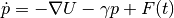where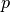is the momentum vector of the set of atoms, interacting via the potential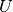,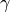is the damping coefficient and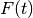is the stochastic force. The random forceis a Gaussian white noise: to enforce the classical fluctuation-dissipation theorem, its autocorrelation spectrum is given by :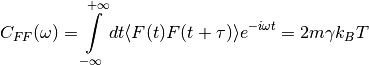where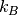is the Boltzmann constant and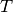the temperature.

### Quantum Thermal Bath (QTB)¶

The Quantum Thermal Bath uses a generalized Langevin equation in order to approximate nuclear quantum effects [Dam] . In QTB dynamics, the stochastic force is no longer a white noise but is colored according to the following formula :

(2)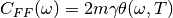with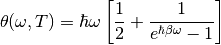where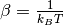and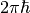is the Planck constant. The function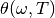describes the energy of a quantum harmonic oscillators of angular frequency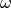at a temperature. The colored random force allows approximating zero-point energy contributions to the equilibrium properties of the system. The QTB method is known to lead to qualitatively good results [Bri] but as many semi-classical methods, it suffers from zero-point energy leakage (ZPEL) [Hern].

The Adaptive Quantum Thermal Bath is an extension of the QTB method, designed to eliminate the zero-point energy leakage by enforcing the energy distribution prescribed by the quantum fluctuation-dissipation theorem for each degree of freedom and each frequency, all along the trajectories [Man] .

In practice, this is done by minimizing the fluctuation-dissipation spectrum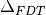defined as:

(3)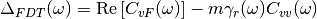where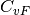is the velocity random force cross-correlation spectrum,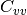the velocity autocorrelation spectrum and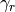a set of damping coefficients dependent (or not) on the frequency.

This minimization is carried out on the fly during the QTB simulation by dissymetrizing the system-bath coupling coefficients corresponding to the damping force (dissipation) and to the random force (energy injection). This can be done either by directly modifying the random force spectrumwith frequency dependent damping term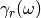(adQTB-r variant) or by modifying the memory kernel of the dissipative force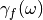within the framework of a non-Markovian generalized Langevin equation (adQTB-f variant).

The coefficientsor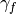are slowly adjusted with a first-order differential equation and an adaptation coefficient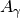:

(4)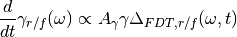during a preliminary “adaptation time” until they reach convergence. Observables are then computed while the adaptive process is kept active. Further information and precise implementation details can be found in ref. [Man].

Two implementations are currently available in PaPIM:

In this variant, the dissipation kernel is left unchanged, i.e.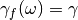while the random force is modified according to a frequency-dependent set of damping coefficientsto satisfy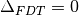(eq. (3)):

(5)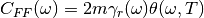This method is applicable only if the initial damping coefficientis large enough to compensate effects of a possible zero-point energy leakage.

In this approach, the random force is not modified, i.e.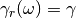and remains the same as in the standard QTB method (eq. (2))) but the dissipation term is not described by a viscous damping term anymore (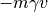) but corresponds to a non-Markovian dissipative force. This leads to the following generalized Langevin equation:

(6)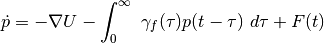In order to avoid solving with brute force this integro-differential equation, the dissipative memory kernel is expressed as a sum of equally spaced (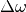) lorentzian functions of width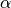:

(7)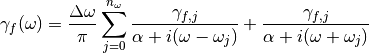The parameterare then modified to satisfy(eq. (3)).

## Input file¶

To run PaPIM using one of the Langevin methods, one must set the parameter sampling_type in the sampling section to one of the following values:

• classical_langevin
• qtb
In this case the parameters n_equilibration_steps and n_mc_steps are ignored and the section langevin is read.

The section langevin must specify the following parameters:
• dt : time step of the Langevin dynamics (REAL)
• lgv_nsteps : number of Langevin steps between each IVR sample (INTEGER)
• lgv_nsteps_therm : number of thermalization steps (INTEGER)
• integrator : integration method (two splitting methods are currently implemented: BAOAB, ABOBA (see reference [Lei] )) (STRING, default=“ABOBA”)
• damping : base damping coefficient for production runs (in eq. :eq:eqLGV) (REAL)
• damping_therm : base damping coefficient for thermalization (in eq. :eq:eqLGV) (REAL)
• qtb_frequency_cutoff : cutoff frequency for the QTB kernel (REAL)
• adqtb_agammas : (Only for adqtbr and adqtbf) adaptation speed coefficient for adQTB (in eq. (4))(REAL)
• adqtb_alpha : (Only for adqtbf) Width of the lorentzian used to represent the dissipative kernel(in eq. (7)) (REAL)
• write_spectra : write average random force autocorrelation function ff, velocity autocorrelation function vv and velocity random force cross-correlation function vf spectra (LOGICAL, default=.FALSE.)
• write_trajectories : write Langevin trajectories in x,y,z,px,py,pz format (LOGICAL, default=.FALSE.)

Remark: all physical quantities are specified in Hartree atomic units.

## Output files¶

The Langevin module is plugged to the IVR subroutines and thus can output the same correlation functions as the classical MC sampling. Additionally, it can write the Langevin trajectories and spectra obtained directly from them.

### Langevin trajectories¶

If the parameter write_trajectories of the langevin section of the input file is set to TRUE, Langevin trajectories are saved. Trajectory files follow the following format:

num_of_atoms

At_symbol(1)   X   Y   Z   Px  Py  Pz
At_symbol(2)   X   Y   Z   Px  Py  Pz
.
.
At_symbol(n)   X   Y   Z   Px  Py  Pz
num_of_atoms

At_symbol(1)   X   Y   Z   Px  Py  Pz
At_symbol(2)   X   Y   Z   Px  Py  Pz
.
.
At_symbol(n)   X   Y   Z   Px  Py  Pz
.
.
.


This corresponds to an extended XYZ format with information on momenta. It is readable by visualization software such as VMD to display the trajectories.

The module outputs multiple trajectory files depending on the number of independent trajectories (blocks) and the number of MPI processes. The naming follows the rules:

• xp.traj.xyz for 1 block and 1 process
• xp_proci.traj.xyz for 1 block and multiple processes
• xp_proci_blockj.traj.xyz for multiple blocks and processes

### QTB analysis files¶

In addition to the trajectories, several files can be edited during the simulations. They are useful to carefully check the convergence of the adaptive QTB, notably by calculating(eq. (3)).

• ff_vv_vf_spectra.out spectra of random force and velocity autocorrelation and random force velocity cross-correlation functions (in atomic units)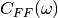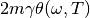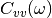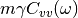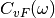• gamas.out (for adQTB-r and adQTB-f only) final set of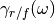optimized during the adaptive procedure (in atomic units)## Tests on implemented potentials¶

### OH anharmonic potential¶

The classical Langevin has been tested on the OH anharmonic potential. The left panel of Figure Fig. 3 shows time correlation functions obtained with IVR using initial conditions sampled from classical (Boltzmann) Monte Carlo and from classical Langevin. Its right panel shows the corresponding spectra obtained by Fourier transform.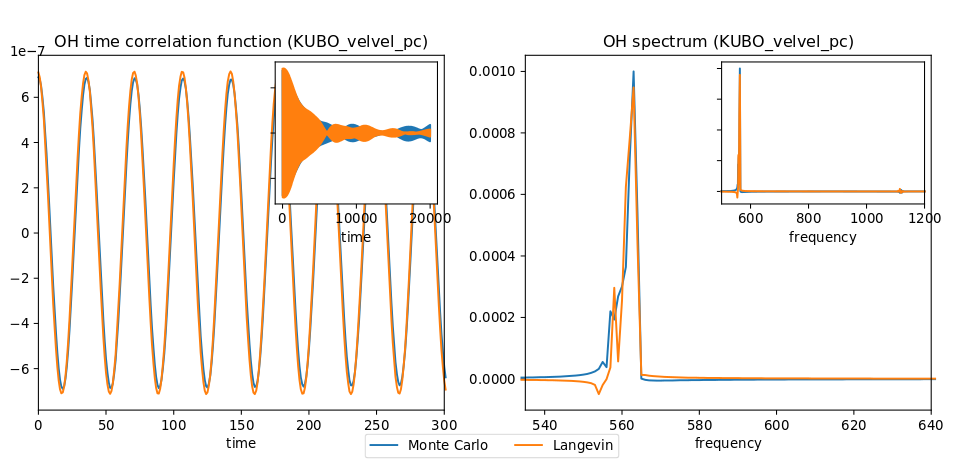Fig. 3 Left panel: OH time correlation function using IVR with initial conditions sampled from MC and from Langevin. Right panel: corresponding spectra obtained by FFT.

### Lennard-Jones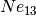cluster¶

A Lennard-Jones potential has been implemented in LennardJonesPot.f90 with the following pair potential:

(8)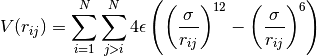A confining pair potential (useful in the cases of small clusters) can be added to eq. (8). A 4th order polynomial is used for distances greater than a chosen distance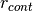:

(9)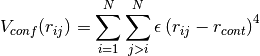Parameters for this potential are specified in an external text file. The file name is given in the input file using the parameter lennard_jones_parameters in section system. The parameters to specify are:

• epsil : depth of the potential well(in Kelvin) (eq. (8))
• sigma : distance for which the potential cancels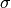(in Å) (eq. (8))
• r_cont : minimum distance for which a confining potentialdefined in eq. (9) is applied (in Å)

The QTB and both adaptive methods were tested on a Ne13 cluster in order to reproduce results from reference [Man]. The Lennard-Jones parameters which have been used are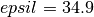,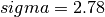and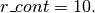5 runs of 8000 steps with 16000 initial time steps are used with all four methods (Langevin, QTB, adQTB-r,adQTB-f). Damping term is set to 5.0e-5 atomic units and adaptive coefficientsandfor adQTB-f to 5.0e-6 atomic units. Pair correlation function is then computed from the trajectories output with a Python script compute_g2r.py. Results are shown in figure Fig. 4 and are in agreement with the ones of ref. [Man].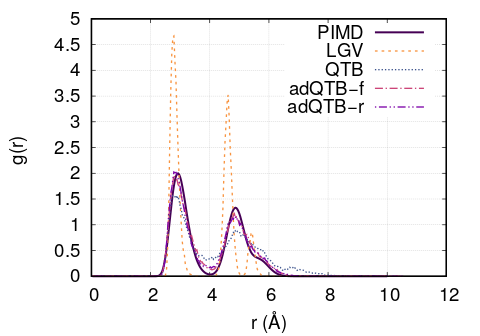Fig. 4 Pair correlation function of Ne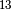cluster obtained with Langevin, QTB, adQTB-r and adQTB-f implemented with Langevin module in PaPIM. Reference curve calculated with Path Integral Molecular Dynamics (PIMD)

In this particular case, adaptive QTB leads to significantly better results than both classical Langevin and QTB when comparing them to the reference results obtained with PIMD (Path Integral Molecular Dynamics).

## Implementation¶

Langevin module is built with the fewest modifications possible in the main and previous code of PaPIM. The main program of the sampler is in the file langevin.f90. It is structured in the same fashion as the existing samplers (PIM.f90 and ClassMC.f90) and only provides the subroutine langevin_sampling to the main program.

### Source files¶

The Langevin module is divided in multiple files:

• langevin.f90: contains the Langevin sampler and links the main code with the other files of the module
• langevin_integrator.f90: subroutines to integrate Langevin equations
• langevin_analysis.f90: spectral analysis tools for Langevin and (ad)QTB trajectories
• qtb_random.f90: generation of QTB colored noise and adaptation subroutines for adQTB

### Other modifications¶

Some other routines have been modified during the implementation of Langevin module.

• PaPIM.f90: main code ; add calls to Langevin module
• GlobType.f90: add declarations for Langevin
• ReadFiles.f90: read input files

## Compiling¶

A Fortran 90/95 compiler with MPI wrapper is required for successful compilation of the code. Although the correlation function subroutines are serial, the remaining code is parallelized so MPI wrappers have to be used. The code must be compiled using the FFTW library. Quantum correlation subroutines within PIM_qtb modules are compiled by executing the command make in the ./source directory. The same make command generates a PaPIM.exe executable for testing of the correlation functions.

## Testing¶

For PIM_qtb test purposes the numdiff package is used for automatic comparison purposes and should be made available before running the tests, otherwise the diff command will be used automatically instead but the user is warned that the test might fail due to numerical differences. The user is advised to download and install numdiff from here. Tests and corresponding reference values are located in sub-directories ./tests/xxx, where xxx stands for oh and lj systems. lj tests also requires a Python distribution. Before running the tests the code has to be properly compiled by running the make command in the ./source sub-directory:

Tests can be executed automatically by running the command in the ./tests sub-directory : #. ./test+lgv.sh for tests on OH bonds compared to previous classical implementation #. ./test_lj.sh for tests on a Ne:. All test are executed on one processor core. Due to small numerical discrepancies between generated outputs and reference values which can cause the tests to fail, the user is advised to manually examine the numerical differences between generated output and the corresponding reference values in case the tests fail.

## Source Code¶

The PIM_qtb module source code is located at: https://gitlab.e-cam2020.eu:10443/thomas.ple/PIM.git (Temporary link).

## Source Code Documentation¶

The documentation can also be compiled by executing the following commands in ./doc/QTB_doc directory with “Sphinx” (documentation tool) python module installed:

sphinx-build -b html source build
make html


The source code documentation can be generated automatically in ./doc sub-directory, html and latex format, by executing the following command in the ./doc directory:

doxygen PIMqcf_doxygen_settings